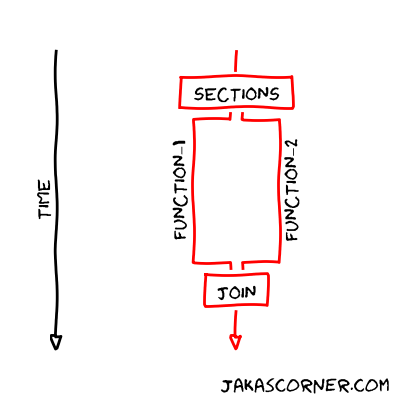We have several tasks which can be executed in parallel. Is it possible to run each task in its own thread?

We already know that this is possible with the C++11 standard library. If we have two functions

we can run them in their own threads:

Is something similar possible with OpenMP? The answer is: yes.

## Sections

The section construct is one way to distribute different tasks to different threads. The syntax of this construct is:

The `parallel` construct creates a team of threads which execute in parallel.

The `sections` construct indicates the start of the construct. It contains several `section` constructs. Each `section` marks the different block, which represents a task. Be aware, the use of singular and plural nouns (`section` / `sections`) is important!

The `sections` construct distributes the blocks/tasks between existing threads. The requirement is that each block must be independent of the other blocks. Then each thread executes one block at a time. Each block is executed only once by one thread.

There are no assumptions about the order of the execution. The distribution of the tasks to the threads is implementation defined. If there are more tasks than threads, some of the threads will execute multiple blocks. If there are more threads than tasks, some of the threads will be idle.

If there is only one `sections` construct inside the `parallel` construct

we can simplify both constructs into the combined construct

The documentation for the `sections` construct is available in the OpenMP specification on the page 65.

## Example

Let us present a simple example.

We have two functions

which print some information. We use the `sleep_for` function to delay the printing between the iterations.

The main function is

At the beginning, the combined construct creates a team of threads. The OpenMP distributes the function calls `function_1();` and `function_2();` between the available threads.

If the number of threads is higher than one, then the program executes the function calls in parallel. If the number of threads is equal to one, than the program executes the function calls sequentially.

The timeline of the program is presented in the picture (if the number of threads is higher than one).The output of the program is

We can clearly see that the functions are executed in parallel.

We can also run the program with only one thread. In this case, the output is